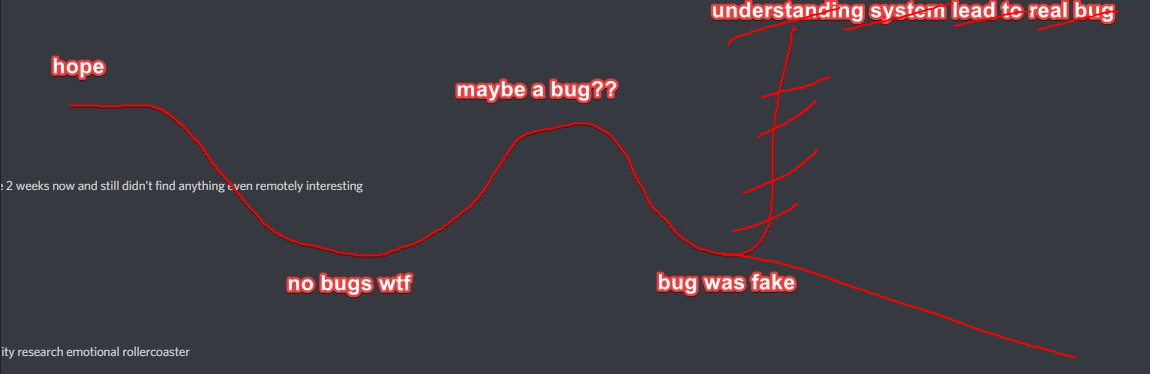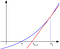# Failed attempt to break Curve

A small project by experience and Killari

We often hear about stories of successful exploits and romantic tales of navigating the Ethereum Dark Forest to save the funds at risk by a thread, but for every uncovered vulnerability, there are likely hundreds of failed attempts at cracking a particular code. We experienced one of these failed attempts while examining the logic of the Curve pool contracts, but even if failed, we found the idea interesting and wanted to share it.The rollercoaster ended well for the safety of users in our story. Credit for the original pic: https://twitter.com/gf_256/status/1365382248988573702?s=20

A few weeks back, I started looking at the Curve logic to try and adapt it to prediction market pools for tokens that have a fixed relative value with ratios different from 1:1, such as for example sports betting outcomes where odds are usually fixed and don’t move much before the game. Navigating through the math of the whitepaper and the contracts to get a good understanding of the Stableswap invariant, I saw that they used the Newton method for finding the root of a function and some memories resurfaced from my freshman years in mathematical methods courses: does this method always converge, and does it always converge to a root? If not, that might have some real security implications for Curve users. Excited about that perspective (reporting a protocol vulnerability to developers and saving funds is a pretty great feeling), I teamed up with Killari, a fellow advanced meme developer, to try to get to the bottom of this. But first, let’s take a few steps back and recall how the protocol works in the first place.

How Curve works: reminder

Curve works similarly to Uniswap except the invariant is not a simple function of a particular pool composition. Assuming a pool with n tokens whose balances are x_i, the quantity that should remain unaffected after a trade is:

The important thing to notice is the D. This is not any random D, it has to be the particular value of D that makes this equation hold with the initial balances loaded. This has to be calculated iteratively: in other words, we’re looking to solve an equation of the form f(D) = 0.

The possibility of a vulnerability

The way the Curve contracts finds the value of D is by using Newton’s method of root finding. This method is widely used because when it converges to a root, it usually does so in very few iterations, and in our case since this is done on the EVM, there are some gas cost implications. The method can be easily understood visually with the animations on the Wikipedia page, what we’re essentially doing is approximating the function by its tangent many times until we find a zero. The iterative formula given an initial value D_ 0 is:

When dealing with real numbers, this sequence can be shown to either converge to a root, or diverge, but it cannot converge to something else than a root. In the Curve pool contracts, for example in the saave pool contract, we can see that Newton method is used with 255 iterations, which should be more than enough to converge if it does converge, and if a root is not reached, the function get_D raises an error and the transaction is reverted. What if however there is convergence before the 255th iteration, and the result is not a root? But wait, didn’t we just say that’s impossible? Well yes, that’s impossible with real numbers, but the contracts are written in Vyper and use 256 bits unsigned integers. So it’s possible to imagine some conditions under which the converge would be to a wrong value of D. Specifically, if at one step of the iteration, f(D_n) < f(D_n+1), the integer division in the iterative step could lead to D_n+1 == D_n, terminating the loop before the actual mathematical convergence to a root.Illustration of a step of Newton’s method, image from Wikimedia Commons

Looking for a way to exploit the potential vulnerability

Our idea was that perhaps this convergence to a wrong value of D could be used to make the spot price diverge from the “expected spot price”, thus allowing an attacker to do risk free arbitrage with a virtual pool. Since there are fees, the new value of D needs to be recalculated each time a trade is made on the AMM, so it might be possible to trigger an invalid D calculation. The attack would look something like this:

1. Find precise pool compositions that make the get_D function converge to a wrong value of D, i.e. one that does not actually yield f(D) = 0.
2. Flash loan the required amount to reach the composition found.
3. Do a swap with that invalid pool.
4. Withdraw liquidity.
5. Do a swap the other way around.
6. Pay back the flash loan.

Perhaps there could be a way to do that such that a net profit would be made from the back and forth trades, extracting tokens from the pool due to the fact that the D is invalid. If found to work, this could be repeated until the pools are emptied.

To figure out if this could work, we translated all the relevant functions from the Curve contracts in a custom Python simulator, which had the advantage of being easy to do since Vyper is pretty much just Python to begin with. Starting from the current balances (and getting a bunch of headaches making sure we got the number of decimals right for each type of Token, cToken or Token with increased precision), we tried adding random amounts of tokens to the pools with the condition that the pools shouldn’t be too unbalanced, and ran multi-threaded processes until we found balances that returned invalid values of D. Our first moment of excitation was when we saw that it was indeed possible to reach wrong values of D.

For this pool composition, we tried all possible combinations of trades (DAI <> USDC, DAI <> USDT, etc) with a prescribed trade amount after adding liquidity, and then after removing it, and iterated until we could find situations in which we got more money out than we put in. After a bunch of false positives that ended up not working in a local mainnet fork, followed by debugging our own simulator (the irony of debugging a codebase to simulate the exploit of another codebase is not lost on us), we ended up not finding anything, even when letting our workstations run a bunch of searches in parallel overnight.

But we did find pool compositions that led to get_D returning an invalid value of D, and were able to confirm that on our mainnet fork. Surely, there has to be a way that this could be exploited right?

Why it (probably) never works

When examining our results, we looked more closely at how invalid was the value of D exactly. Sure, f(D) was not 0, but how far was it from it? As it turns out, at least in our simulation results, the deviation was always completely tiny, because when compared to what should be the “correct” D for that pool composition, the invalid D was actually very close. We suspect that integers math saves the day by making this tiny difference not matter at all when calculating a trade amount. So what caused the invalid D calculation in the first place could nullify its impact. Two wrongs would make a right! Or it could be that the fees are significantly bigger, making the effect overall negligible.

Using custom functions to imitate the behavior of the Curve invariant with real numbers, with the help of SciPy functions for root finding, we looked at the kind of deviations of D that would be needed for the spot price to deviate significantly (> 1%) fromm the “expected” spot price with the invariant at a given pool composition. Our results indicate that the invalid D would have to have a value 10 to 30% higher compared to the correct one. This difference is many orders of magnitude beyond the kind of differences that we could find. So at least based on our results, it would appear that there is no way to extract value from the Curve pools using this angle of attack.

Another failed angle of attack

The USDT pool or other early Curve pools do not raise any error if we go beyond the 255 iterations. Another possibility would be to find pools composition that make the Newton method oscillate between two values of D. such that we don’t converge to anything. What we would need would be for the iteration to yield D2 == D0, and then it would bounce back and forth between D0, D1, where D0 is defined as the sum of pool balances in the Curve codebase.

Looking for 3-token pool compositions such that D2 == D0 looks like this:

1. Do the math to get closed form expressions of D0 and D2 given pool compositions (x, y, z)
2. Solve D2(x,y,z) — D0(x,y,z) = 0 for (x,y,z)

We did that using a scipy.optimize function and found a value where it works. But fortunately for the users of the early Curve pools (and unfortunately for us because no bug bounty!), the only value of (x,y,z) that verifies that equation is the case of perfectly balanced pools, where as it turns out, the initial guess D0 = x + y + z is also the valid value of D, so this doesn’t lead us anywhere.

Closing word

This was a fun little project for us, and even though we would have loved the excitement of reporting a protocol vulnerability, we’re glad that we failed, which means the Curve pools are still safe from our idea! That being said, we only explored the parameter space with our personal workstations, so even though we doubt it, there could be some specific values out there that do the trick of deviating D by at least 10%. If you have access to a supercluster to mine such value, be sure to report it to the Curve security team :)

Note

This blog post was published after discussing its content with the Curve security team at security@curve.fi and with their authorization.

Our social media accounts:

experience — experience#2376 on Discord

Killari — Killari#5195 on Discord, @Qhuesten on Twitter